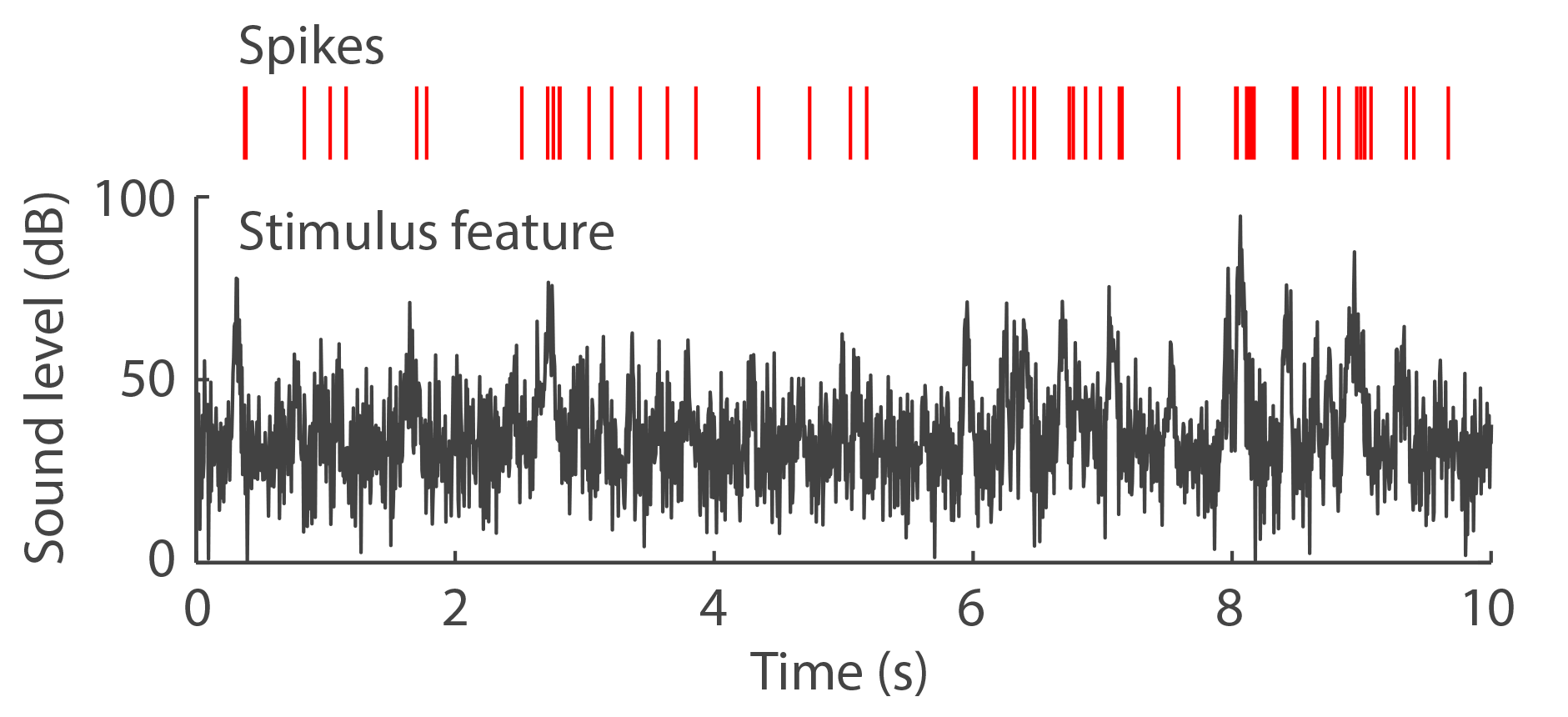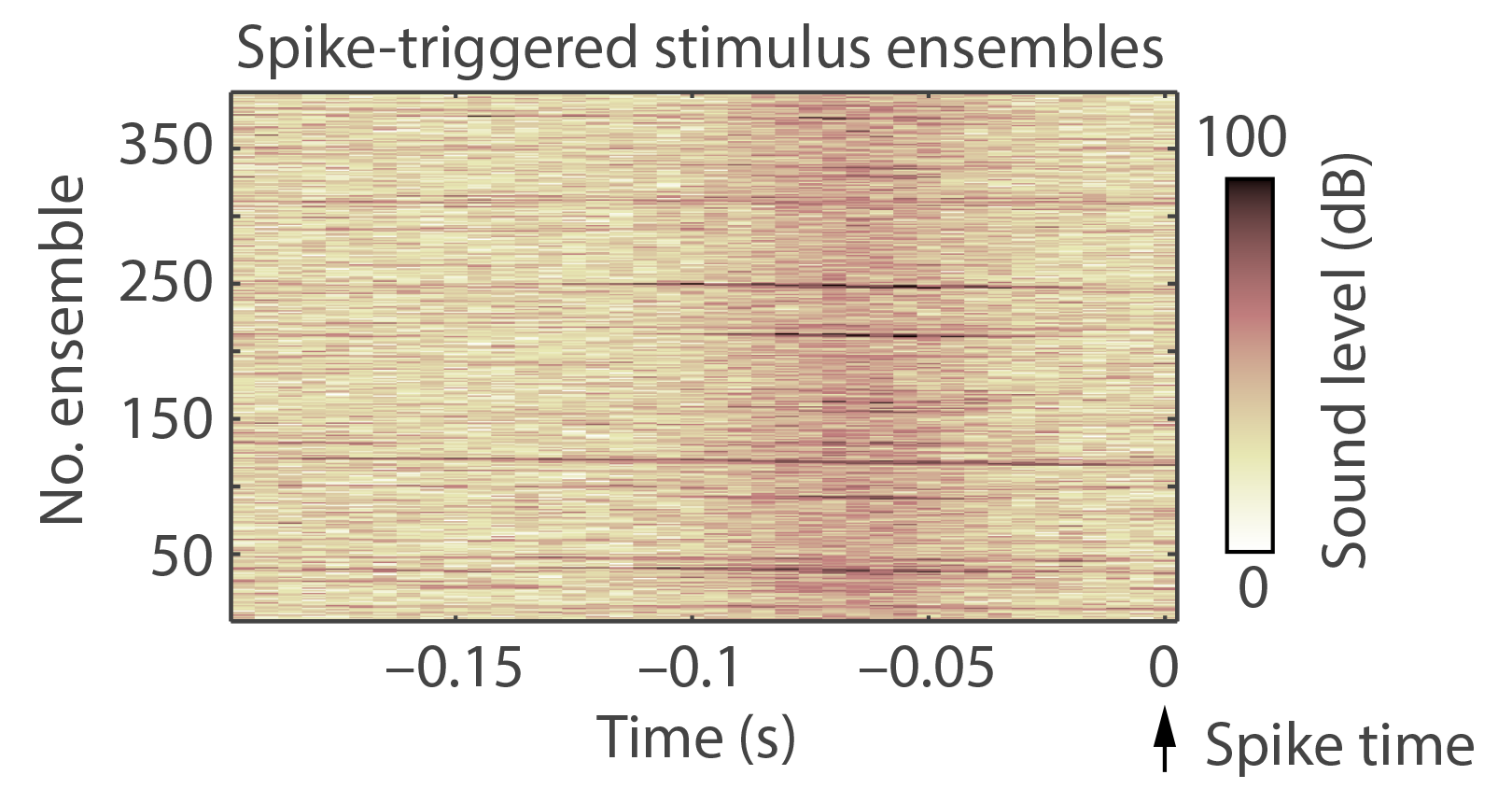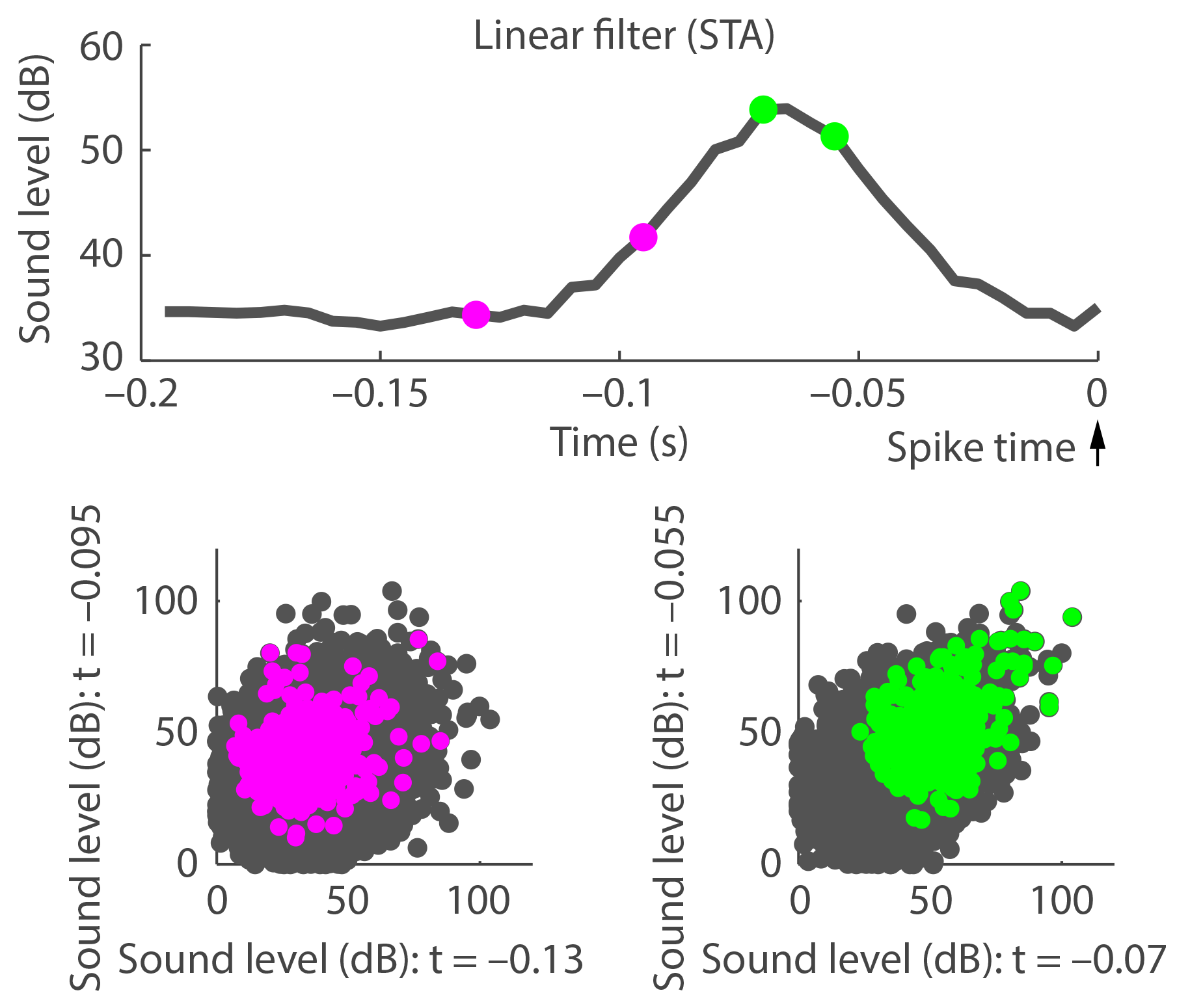Linear-nonlinear Poisson (LNP) model

Neural response properties are often characterized using receptive fields. In the current context, a receptive field describes the stimulus properties to which a neuron is particularly sensitive. Linear-nonlinear Poisson (LNP) models aim to characterize the functional response properties of neurons using stochastic stimuli (i.e., stimuli changing randomly in some attribute(s)). LNP models have been used to investigate how neurons adapt to stimulus statistics (Rabinowitz et al. 2011) and how stimulus input-to- neural output functions change depending on the phase of neural oscillations (Kayser et al. 2015). Based on an LNP model we can predict spike times given a stimulus.

The current tutorial is meant to provide a simple description of the linear-nonlinear Poisson model to get an individual acquainted with the general idea. More detailed tutorials can be found in the paper by Schwartz et al. (2006) and the book chapter by Simoncelli et al. (2004). These authors also provide matlab code for the spike-triggered analysis. The LNP model consists of 3 steps: (1) estimating linear filters, (2) a nonlinearity, transforming the output of these filter(s) to an instantaneous firing rate, and (3) a Poisson spike generation process based on the output from the nonlinear transformation. Matlab code for the current tutorial is provided below.

Simulated data

Imagine a study in which spiking activity is recorded in response to a sound that randomly changes in sound level (i.e., volume/intensity).For this simulation, a vector containing random sound levels was generated (100 s duration, 200 Hz sampling frequency; with a mean sound level of 30 dB). Spikes were generated at random time points and a Gaussian function (i.e., a sound level increase) was added to the vector just prior to each spike. I added the Gaussian functions in order to introduce a consistent change in the stimulus that is related to spiking. It will be assumed that something in the stimulus elicited a spike (or more spikes). The spike-triggered analysis can tell us what this something is (here we already know). 10 seconds of the stimulus' sound levels and the simulated spikes are displayed in the figure on the left.

Stimulus ensemblesCritically for spike-triggered analyses is the term "stimulus ensemble". A stimulus ensemble is a matrix (here a 1-dimensional matrix) containing stimulus information over a certain period of time. For the current tutorial, the time window was 0.2 seconds (or 40 points) long and the 1D matrix contained sound levels. A dinstinction is made into "stimulus ensemble" and "spike-triggered stimulus ensemble". The former could be any stimulus matrix and the full pool of stimulus ensembles comes from slidding the time window sample-by-sample over the whole stimulus and extracting a stimulus matrix each time (here leading to ~20.000 ensembles). Spike-triggered stimulus ensembles are the subset of ensembles from the full pool that occurred directly prior to a spike. In practice a short delay between spike-triggered stimulus ensemble and spike is used to account for spike latency (this was neglected here). The 391 spike-triggered ensembles from the current simulation are displayed in the figure on the right. The increase in sound level just prior to the time of a spike is clearly visible.

Linear filter estimation: Spike-triggered average (Step 1)

What function describes the difference between stimulus ensembles that did not elicit a spike versus the spike-triggered stimulus ensembles?We would like to estimate a linear filter representing the stimulus properties a neuron cares about. The simplest deviation between stimulus ensembles and spike-triggered ensembles is a change in the mean. The spike-triggered average (STA) reflects a linear function by which changes in mean stimulus level can be characterized (spike-triggered covariance, STC, can be used to characterize changes in variance).

The STA is calculated by averaging across all spike-triggered ensembles and then subtracting the mean across time points (centering the STA on zero). The STA (non-mean subtracted) is displayed in the figure on the right. Oftentimes, two of the multiple stimulus dimensions are displayed (shown below the STA). At two different time points (marked with a circle) the two stimulus levels of one ensemble are plotted as one point in 2D space, where the stimulus level at one time point is plotted along the x-axis and the stimulus level at the other time point along the y-axis. This is repeated for all stimulus ensembles (gray) and all spike-triggered ensembles (colored). Depending on the two time points selected one can see a deviation from the mean (right plot). The linear filter (STA) essentially reflects the receptive field of the neuron (linear filter calculation was done using this matlab code).

Characterization of the nonlinearity (Step 2)

In the LNP model, the firing rate of a neuron is calculated by a nonlinear transformation of the linear filter response/output (hereafter output).The output of the linear filter is calculated as the dot product between the filter k and a stimulus ensemble s (k x s, with x being the dot product). The filter output is then calculated for each stimulus ensemble, followed by the calculation of the probability of the output to occur given the stimulus ensembles. This probability is simply the proportion of dot products (or histogram; see figure on the left), describing the likelihood of a specific linear filter output. Subsequently, the probability of the output given the spike-triggered stimulus ensembles is calculated (red). An empirical, non-parametric estimate of the nonlinearity is based on these two distributions and calculated by dividing the probability distribition of the stimulus ensembles by the the probability distribition of the spike-triggered stimulus ensembles. The result is plotted in the right part of the figure (solid line). A different way of characterizing the nonlinearity is by using a parametric function, for example, a linear function with an intercept at zero and which is zero for negative filter outputs (dashed line). Generally, the nonlinearity provides an instantaneous firing rate for each output of the linear filter (calculation of the nonlinearity was done using this matlab code).

Poisson spike generation (Step 3)Having a linear filter and a nonlinearity transforming the filter output to an instantaneous firing rate allows the generation of spike times for a new stimulus with random sound levels (figure on the right; stimulus generation was done as before). That is, we can predict spikes based on the sensitivity of the neuron (i.e., the model) for any stimulus with similar properties.

The pool of stimulus ensembles was calculated for the new stimulus. Hence, we have for each time point (except for the first 0.2 s) a stimulus ensemble (1D matrix), which becomes transformed to an instantaneous firing rate by applying the linear filter and nonlinear transformation (prediction of firing rate was done using this matlab code). The result is an instantaneous firing rate over time (bottom part of the figure). The time course of instantaneous firing rate can be converted to a spike train using a Poisson process (spikes were generated using this matlab code).

The matlab scripts used to do the simulations can be downloaded here. Please only use under under GNU license. No warranty can be provided. Self-check for errors.